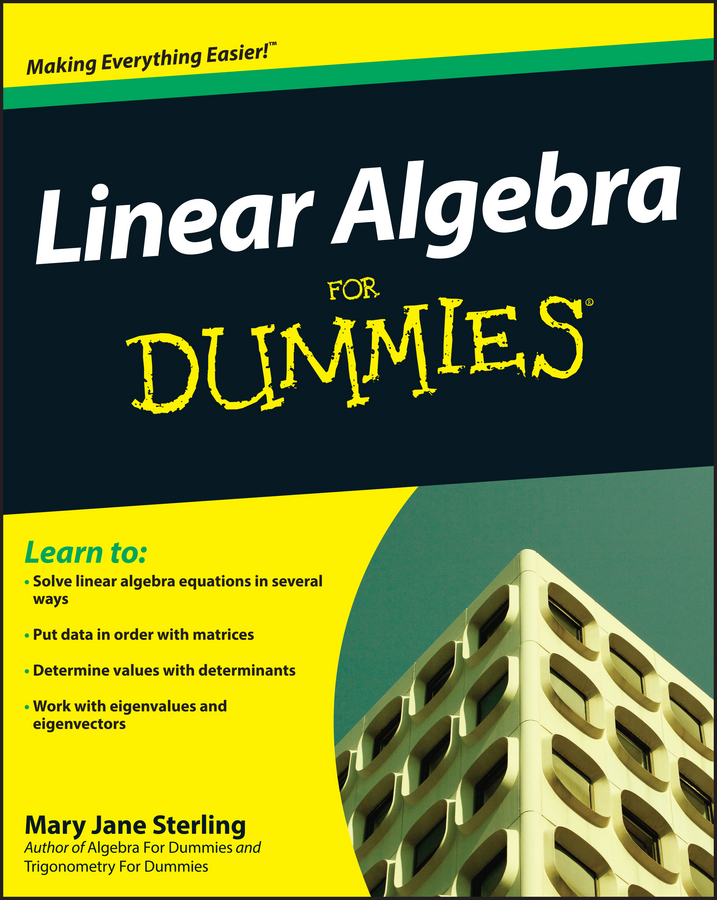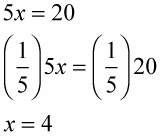##### Linear Algebra For DummiesWhen solving an equation in algebra, you will often use an opposite operation such as additive inverse or multiplicative inverse (reciprocal) to work your way toward the answer. You have to undo operations that have been done to the variable. The opposite of an operation is another operation that gets you back where you started. This is used primarily to get rid of numbers that are combined with a variable so you can solve for the variable in an equation.

When you need to use the opposite of basic operations — addition, subtraction, multiplication, and division — you need to remember how the additive inverse and multiplicative inverse work:

• The additive inverse is the number with the opposite sign. So −3 is the additive inverse of 3, and 16 is the additive inverse of −16. Use these if 3 or 16 is being added to a variable, and you want to get the variable alone; this is used when solving an equation for the value of the variable.

For example, if you have the problem 4 + x = 10, you have to use an opposite operation to solve for the variable x.

4 + x = 10

(4 – 4) + x = (10 – 4)

0 + x = 6

x = 6

• The multiplicative inverse is also called the reciprocal. The reciprocal is the original number written as the bottom of a fraction with a 1 on the top. So 1/2 is the reciprocal of 2. If a number starts out as a fraction, its reciprocal is just that number written upside-down. So the reciprocal of 4/7 is 7/4. Similarly, the reciprocal of 1/25 is 25/1, or simply 25.

You use a reciprocal if a number multiplies or divides a variable; it gets the variable alone so it can be solved for. For example, if you have the problem 5x = 20, you have to use an opposite operation to solve for the variable x.In algebra, you use more than just the basic mathematical operations, so when you need to solve an algebra problem using opposite operations, remember this list:

• The opposite of addition is subtraction.

• The opposite of subtraction is addition.

• The opposite of multiplication is division.

• The opposite of division is multiplication.

• The opposite of taking a square root is squaring (multiplying a value by itself).

• The opposite of squaring is taking the square root.

• The opposite of cubing is taking the cube root.